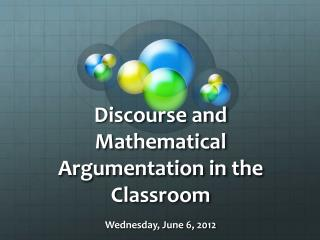Download PresentationDiscourse and Mathematical Argumentation in the Classroom

# Discourse and Mathematical Argumentation in the Classroom

Download Presentation## Discourse and Mathematical Argumentation in the Classroom

- - - - - - - - - - - - - - - - - - - - - - - - - - - E N D - - - - - - - - - - - - - - - - - - - - - - - - - - -
##### Presentation Transcript

1. Discourse and Mathematical Argumentation in the Classroom Wednesday, June 6, 2012

2. Computing seems to be an instructional priority despite the fact that the field of mathematics goes far beyond procedures. • At its core, mathematics involves exploring, arguing, and justifying. • We need to find a way to go beyond procedures in order to teach for conceptual understanding.

3. 8 Standards for Mathematical Practice • Make sense of problems and persevere in solving them. • Reason abstractly and quantitatively. • Construct viable arguments and critique the reasoning of others. • Model with mathematics. • Use appropriate tools strategically. • Attend to precision. • Look for and make use of structure. • Look for and express regularity in repeated reasoning.

4. Discourse that Promotes Conceptual Understanding by ElhamKazemi • 3 things that are clearer to you regarding discourse, mathematical argumentation, and sociomathematical norms • 2 connections to your own classroom • 1 question or point you would like to discuss further

5. Kazemi’s Main Points

6. Discuss in pairs… Kazemi wrote that “press for learning was measured by the degree to which teachers • emphasized students’ effort • focused on learning and understanding • supported students’ autonomy • emphasized reasoning more than producing correct answers.”

7. What does mathematical argumentation look like in a classroom? • How can we incorporate mathematical argumentation into our lessons?

8. My Research Study • Mathematical Context: Arithmetic Properties • Goals: • Identify and describe the characteristics of students’ mathematical arguments related to the arithmetic properties • Quantify growth when instruction promoted mathematical argumentation • Two Fourth-Grade Classrooms • One had more opportunities for argumentation and an emphasis on explaining and exploring. • One followed the traditional textbook and worksheets.

9. Depth of Knowledge Traditional Textbook Tasks Modified-Lesson Tasks If you take any number and multiply it by zero, you will always get a number larger than 100. Do you agree or disagree? Explain. 5 + 1 = _____ + 5 “What should go in the blank so that the following number sentence is true? Who can explain why their answer is correct?”

10. Teacher Moves and Sociomathematical Norms Language Frames (Ross, Fisher, & Frey, 2009) • I believe _____ (statement) because _____ (justification). • Based on _____, I think _____. • I noticed _____, when _____. • I disagree with ____ because ____. Sentence Starters (Loper and Baker, 2009) • What do you think? • What is your claim? • Why do you think that? • What is your evidence? • My claim is…

11. What teacher moves do you notice?

12. My Teacher Moves I had 23 teacher moves but only six of them occurred before mathematical arguments: • repeats or restates • invites ideas or observations • invites students to agree or judge • prompts for justification, explanation, or convincing • prompts for elaboration or clarification • gives a false claim

13. Facts about my Teacher Moves • The most commonly used teacher move (repeating or restating) did not occur before many arguments. • The teacher move that most frequently occurred before a student argument was a prompt for justification or explanation (about 65%) even though it was not a common teacher move overall (occurring only about 7 % of the time).

14. Mathematical Errors • What are some ideas about teacher moves for a mathematical error introduced by a student? • What have you done in the past? • What are your ideas about introducing false claims?

15. What are the students’ reactions to my false claim?What could I have done next to follow up?

16. Did including mathematical argumentation make a difference in student learning? Pre- and Post-Assessment Data Individual interview assessments with students in both classrooms focused on: • Correctness • Computational vs. Number Pattern Strategies • Recognition of a Property

17. Correctness

18. Computation vs. Number Pattern Strategies

19. Pre- and Post- Assessment Results Summary Some students in both classrooms showed improvement on the assessment. Students in the focus classroom applied their understanding of properties more frequently than in the contextual classroom. Students in the focus classroom applied more number pattern strategies to the assessment tasks.

20. Discussion Points • What are the benefits and disadvantages of including mathematical argumentation? What could be done to alleviate the disadvantages? • How can you include mathematical argumentation in your knowledge package topic?

21. Depth of Knowledge and Standards for Mathematical Practice • In order to teach at higher levels of the DOK framework, we need to be including mathematical argumentation and justification. • Mathematical argumentation is one of the 8 Standards for Mathematical Practice. • What are the characteristics of tasks aligned with standards for mathematical practice, high DOK levels, and mathematical argumentation? http://vimeo.com/30924981

22. Homework • Read What exactly do “fewer, clearer, and higher standards” really look like in the classroom? Using a cognitive rigor matrix to analyze curriculum, plan lessons, and implement assessment. Karin K. Hess, Dennis Carlock, Ben Jones, John R. Walkup • Reflection